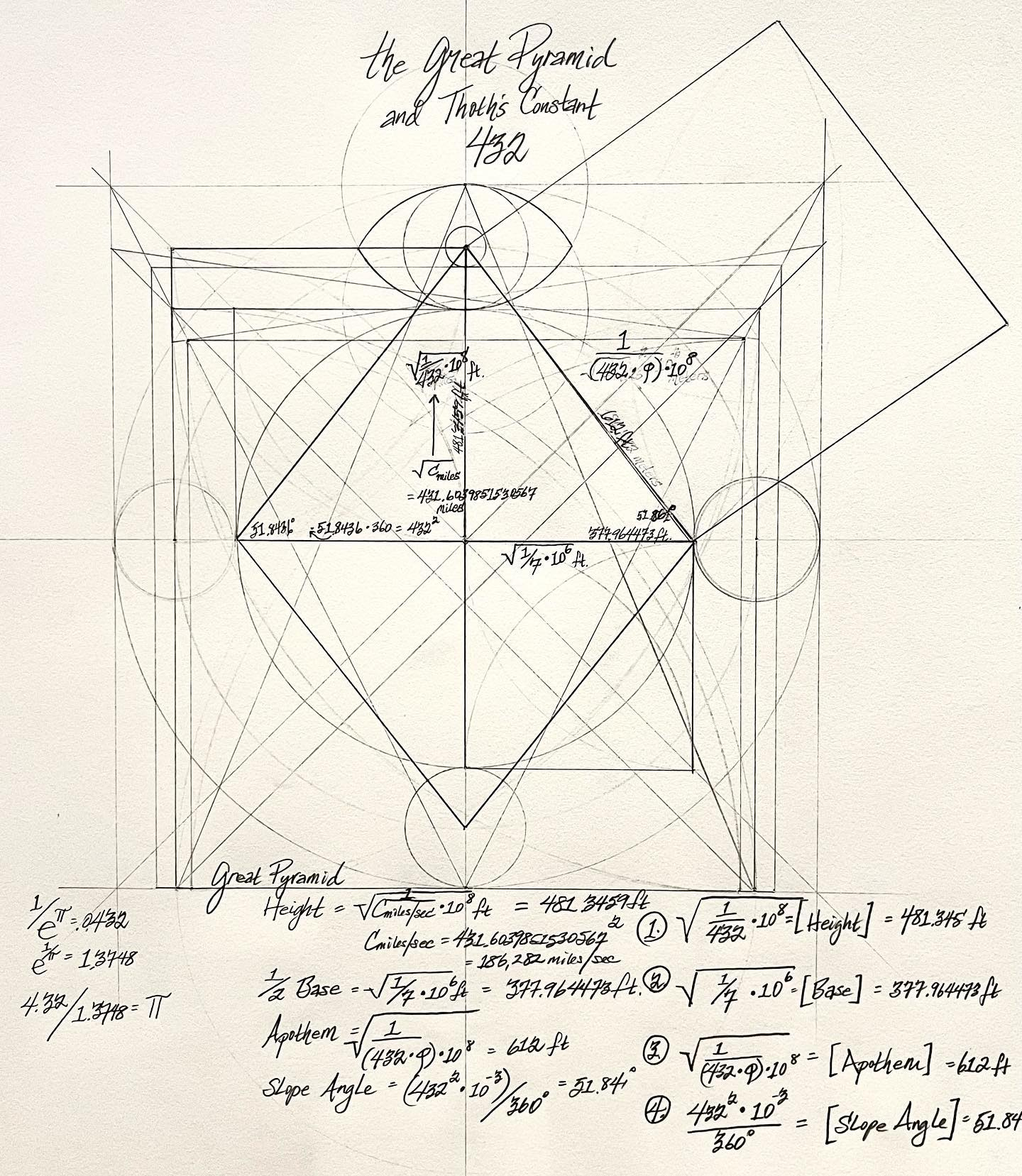Is there only ONE value (432) that matters when analyzing the Proportional Dimensions of the Great Pyramid? {Alan Green‘s research has already established that the Architects of the Great Pyramid used all three measurement systems (Foot, Cubit and Meter) in its construction and fundamental proportions}.

432 is also the only value that ‘marries’ Euler and π…..The Great Pyramid and Thoth’s Constant of 432….(references: φ = .6180339887; Φ = 1.6180339887; π = 3.1415926; e = 2.7182818). 1. The Height: {((1/432)*10^8)^1/2} = 481.345 feet 2. 1/2 Base: {(1/(432.624/φ*10^2°)*10^8)}^1/2 = 377.964473 feet 3. The Apothem: {(1/(432φ))*10^8}^1/2 = 612 feet 4. The Slope Angle: {(432^2)*10^-3}°/360° = 51.84° All are derivations of the Pythagorean Tetractys Number 432.

Where else do we see 432 showing up in mathematical constants?
1. e^π = 1/.0432 2. e^1/π = 1.3748 3. 4.32/1.3748 ≈ π (3.142) This is highly interesting because this means the following: 1. (e^π)*10^4 ≈ The Height of the Great Pyramid = 481 feet; (or precisely 481.0477 feet))
2. {((e^π)*10^4)/((φ*10^2°)*10^8)}^1/2 = 1/2 The Base of the Great Pyramid = 377.96 feet (exactly 378.176 feet)
3. {(1/(10^4/(e^π))*φ)*10^8)}^1/2 ≈ The Apothem of the Great Pyramid = 612 feet (611.902 feet to be exact))
4. {({(10^4)/(e^π)}^2)*10^-3}°/360° ≈ 51.84° (51.87° to be precise)

What are the Pyramid Architects trying to tell us about the Universal relationship between the mathematical constants Φ, π, e, 360 and 432? Could it be that Φπe = {(13.8175/10)-1}*360 = 137.4328, which diameter makes a circle with a Circumference of ≈ 432 (431.758).

Or put differently: {{(Φπe/10)-1}*360π = 432…..Is it any wonder then that 432 is the only whole number that squares to Light Speed in miles/second (C = 186,282 miles/second and it’s square root = 431.6).

Artwork and Analysis by REG.